# Python | Pandas Index.difference()

Python is a great language for doing data analysis, primarily because of the fantastic ecosystem of data-centric python packages. Pandas is one of those packages and makes importing and analyzing data much easier.

Pandas` Index.difference()` function return a new Index with elements from the index that are not in other.
The function automatically sorts the output if sorting is possible.

Syntax: Index.difference(other)

Parameters :
other : Index or array-like

Returns : difference : Index

Example #1: Use `Index.difference()` function to find the set difference of a given Index with an array-like object.

 `# importing pandas as pd ` `import` `pandas as pd ` ` `  `# Creating the Index ` `idx ``=` `pd.Index([``17``, ``69``, ``33``, ``15``, ``19``, ``74``, ``10``, ``5``]) ` ` `  `# Print the Index ` `idx `

Output :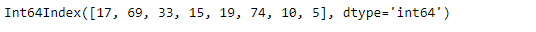Let’s find the set difference of the given Index with an array-like object

 `# find the set difference of this Index  ` `# with the passed array object. ` `idx.difference([``69``, ``33``, ``15``, ``74``, ``19``]) `

Output :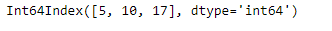As we can see in the output, the function has returned an object which contains only those values which are unique to the idx Index.

Note that the output object has its element sorted in Increasing order.

Example #2: Use `Index.difference()` function to find the set difference of two Indexes.

 `# importing pandas as pd ` `import` `pandas as pd ` ` `  `# Creating the first Index ` `idx1 ``=` `pd.Index([``'Jan'``, ``'Feb'``, ``'Mar'``, ``'Apr'``, ``'May'``, ``'Jun'``, ` `                 ``'Jul'``, ``'Aug'``, ``'Sep'``, ``'Oct'``, ``'Nov'``, ``'Dec'``]) ` ` `  `# Creating the second Index ` `idx2 ``=` `pd.Index([``'May'``, ``'Jun'``, ``'Jul'``, ``'Aug'``]) ` ` `  `# Print the first and second Index ` `print``(idx1, ``"\n"``, idx2) `

Output :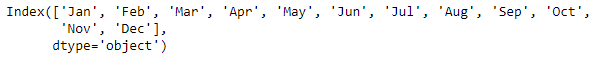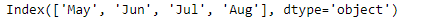Now, let’s find the set difference between two Indexes.

 `# to find the set differnece ` `idx1.difference(idx2) `

Output :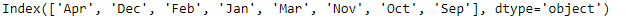The function has returned the set difference of idx1 and idx2. It contains only those values which are unique to idx1 Index. Note that the output is not sorted.

My Personal Notes arrow_drop_upCheck out this Author's contributed articles.

If you like GeeksforGeeks and would like to contribute, you can also write an article using contribute.geeksforgeeks.org or mail your article to contribute@geeksforgeeks.org. See your article appearing on the GeeksforGeeks main page and help other Geeks.

Please Improve this article if you find anything incorrect by clicking on the "Improve Article" button below.

Article Tags :

Be the First to upvote.

Please write to us at contribute@geeksforgeeks.org to report any issue with the above content.